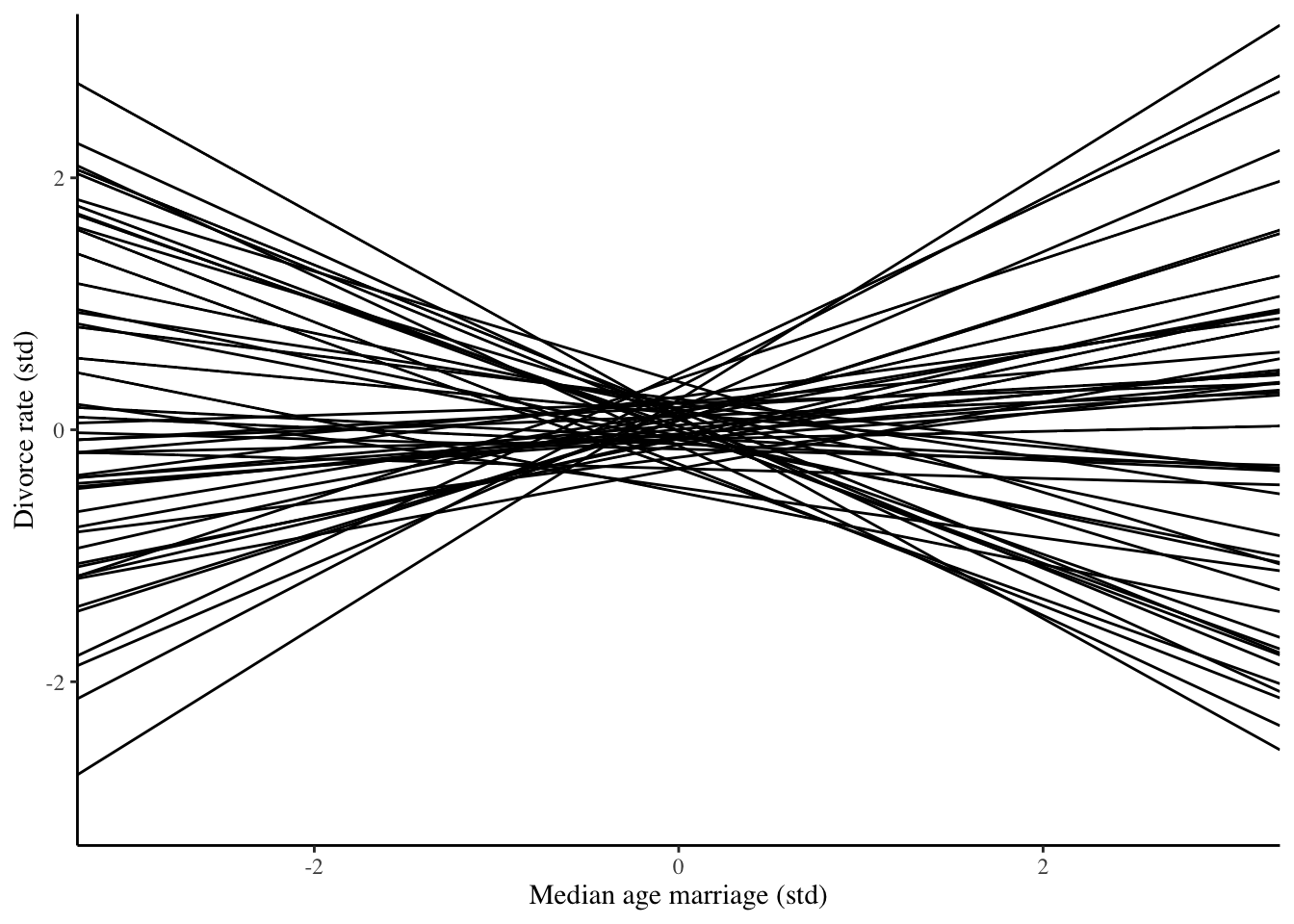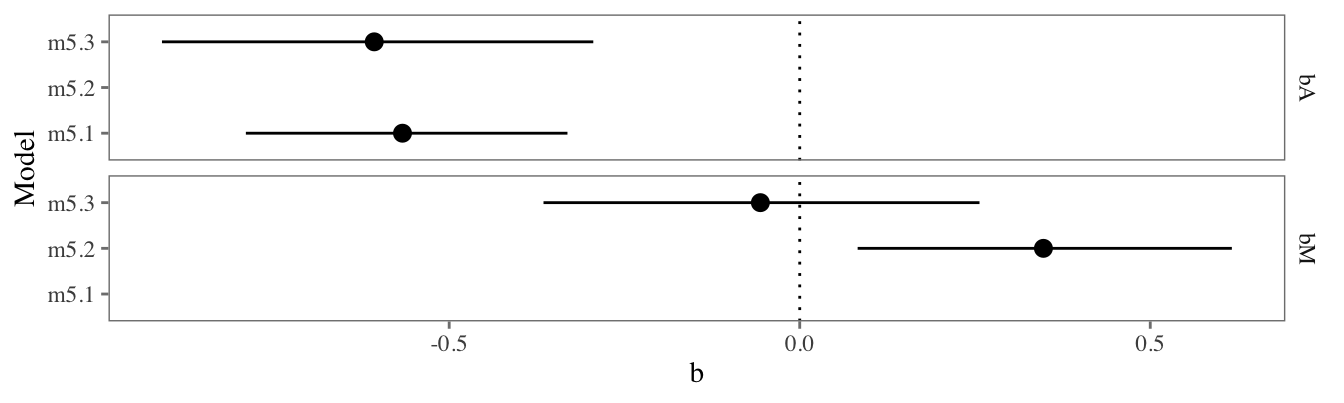# Status

Estimated and checked against the book:

• m5.1
• m5.2
• m5.3
• m5.4
• m5.5
• m5.6
• m5.7
• m5.8
• m5.9

Warning: there are small numerical differences, probably due to the difference between quap and Stan.

# Libraries

library(tidyverse)
library(tidybayes)
library(rstan)
library(patchwork)
options(mc.cores = 4)

# Section 5.1: Spurious association

Plausible regression lines implied by the priors:

We will estimate a series of regression models with a constant $$\alpha$$ and regression coefficients $$\beta_k$$, and these priors:

$\alpha \sim N(0, .2)$ $\beta_k \sim N(0, .5)$

To see if these priors make sense, we can plot a few of the regression lines implied by these priors. To do this, we draw random numbers from the distributions above, and we plot the corresponding regression lines:

a = rnorm(50, 0, .2)
b = rnorm(50, 0, .5)
p <- ggplot()
for (i in 1:50) {
p <- p + geom_abline(slope = b[i], intercept = a[i])
}
p + xlim(-3, 3) + ylim(-3, 3) +
labs(x = 'Median age marriage (std)',
y = 'Divorce rate (std)')stan_program <- '
data {
int<lower=1> n;   // number of observations
int<lower=1> K;   // number of regressors (including constant)
vector[n] Divorce;      // outcome
matrix[n, K] X;   // regressors
}
parameters {
real<lower=0,upper=50> sigma;    // scale
vector[K] b;                     // coefficients (including constant)
}
transformed parameters {
vector[n] mu;                    // location
mu = X * b;
}
model {
Divorce ~ normal(mu, sigma);    // probability model
sigma ~ exponential(1);   // prior for scale
b ~ normal(0, 0.2);    // prior for intercept
for (i in 2:K) {          // priors for coefficients
b[i] ~ normal(0, 0.5);
}
}
generated quantities {
vector[n] yhat;           // predicted outcome
for (i in 1:n) yhat[i] = normal_rng(mu[i], sigma);
}
'

standardize <- function(x) as.vector(scale(x))
WaffleDivorce <- read.csv('data/WaffleDivorce.csv', sep = ';') %>%
mutate(across(c(Divorce, Marriage, MedianAgeMarriage), standardize))

stan_data <- WaffleDivorce %>%
compose_data(K = 2,
y = Divorce,
X = model.matrix(~MedianAgeMarriage, .))

m5.1 <- stan(model_code = stan_program, data = stan_data)

stan_data <- WaffleDivorce %>%
compose_data(K = 2,
y = Divorce,
X = model.matrix(~Marriage, .))
m5.2 <- stan(model_code = stan_program, data = stan_data)

stan_data <- WaffleDivorce %>%
compose_data(K = 3,
y = Divorce,
X = model.matrix(~MedianAgeMarriage + Marriage, .))
m5.3 <- stan(model_code = stan_program, data = stan_data)
res5.1 <- m5.1 %>%
mean_qi %>%
mutate(i = c('Intercept', 'bA'),
Model = 'm5.1')
res5.2 <- m5.2 %>%
mean_qi %>%
mutate(i = c('Intercept', 'bM'),
Model = 'm5.2')
res5.3 <- m5.3 %>%
mean_qi %>%
mutate(i = c('Intercept', 'bA', 'bM'),
Model = 'm5.3')
res <- bind_rows(res5.1, res5.2, res5.3) %>%
filter(i != 'Intercept')

ggplot(res, aes(x = b, y = Model, xmin = .lower, xmax = .upper)) +
geom_vline(xintercept = 0, linetype = 'dotted') +
geom_pointrange() +
facet_grid(i ~ .) +
vincent::theme_vab()Figure 5.5:

datplot <- m5.3 %>%
gather_draws(mu[i]) %>%
mean_qi %>%
rename(Predicted = .value)
datplot$Observed <- WaffleDivorce$Divorce

ggplot(datplot, aes(Observed, Predicted,
ymin = .lower, ymax = .upper)) +
geom_pointrange() +
geom_abline(intercept = 0, slope = 1, linetype = 'dashed') +
labs(x = 'Observed divorce', y = 'Predicted divorce',
title = 'Posterior predictive plot') +
coord_fixed()